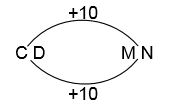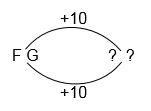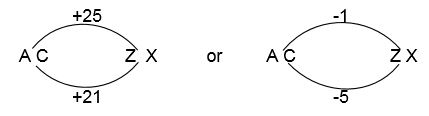# VR - Similarities Between Letter Pairs

Example

These questions use the concept of mathematical series but using letters. The alphabet is used and there is usually a numerical link to find as in the 'letter series' type of question.

C D is to M N as F G is to ...

Firstly, make sure you are familiar with the style of question in the previous section – this is just a variation. Let’s use the same technique to see what the answer should be.The first letter of the first pair is going to be related to the first letter of the second pair. In this case, M is ten letters along the alphabet from C so I have written +10 along the top. Similarly, N is ten letters along the alphabet from D so I have written +10 on the bottom.

Now, simply relate that information to the new set of letters.Counting along the alphabet – and encourage your child to use this rather than just do it in his or her head – we find the answer must be PQ.

If they get used to the question format and are becoming increasingly able to do it mentally, that's fine - but encourage them to have the know-how to cope with a written method that should guarantee a correct answer. Good candidates get the technique right first then apply it mentally, rather than on paper, to save time. Most of these questions follow a very similar format but there can be an occasional odd ones, which the next example will demonstrate.

Example 2

A C is to Z X as D E is to ...

This time, and this is not a very common question, the counting method may not work. Let’s look at the way it would be:Remember to tell your child that you should use the alphabet as if it was a loop – you can go from A back to Z. This makes it an easier – or at least quicker – calculation. However, this sort of question is almost certainly going to be multiple choice and if the following were the options then it’d be impossible through this method.

Possible answers: E D X Z W V W Z V W

The standard method would say it should be C Z but this is not a possible answer. So, look at the alternatives. The alphabet – with the letters highlighted – looks like this:

A B C D E F G H I J K L M N O P Q R S T U V W X Y Z

The symmetry is what we are looking at here. A and Z are at the opposite ends of the alphabet while C and X are three letters in from each end.

Now, look at our second set of letters – D E – and find the letters which are symmetrically opposite.

A B C D E F G H I J K L M N O P Q R S T U V W X Y Z

Given that D is the fourth letter from the start of the alphabet, its symmetrical opposite is W. The opposite of E is V. The answer to the question is therefore W V.